Tamilnadu State Board New Syllabus Samacheer Kalvi 11th Chemistry Guide Pdf Chapter 8 Physical and Chemical Equilibrium Text Book Back Questions and Answers, Notes.

## Tamilnadu Samacheer Kalvi 11th Chemistry Solutions Chapter 8 Physical and Chemical Equilibrium

### 11th Chemistry Guide Physical and Chemical Equilibrium Text Book Back Questions and Answers

Textbook Evaluation:

Question 1.
If Kb and Kf for a reversible reaction are 0.8 × 10-5 and 1.6 × 10-4 respectively, the value of the equilibrium constant is,
a) 20
b) 0.2 × 10-1
c) 0.05
d) None of these
a) 20

Question 2.
At a given temperature and pressure, the equilibrium constant values for the equilibria
3A2 + B2 + 2Cr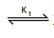2A3BC and
A3BC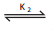$$\frac{3}{2}$$ [A2] + $$\frac{1}{2}$$ B2 +C
The relation between K1 and K2 is
a) K1 = $$\frac{1}{\sqrt{\mathrm{K}_{2}}}$$

b)K2 = $$\mathrm{K}_{1}^{-1 / 2}$$

C) K12 = 2K2

d) $$\frac{\mathrm{K}_{1}}{2}$$ = K2
b)K2 = $$\mathrm{K}_{1}^{-1 / 2}$$

Question 3.
The equilibrium constant for a reaction at room temperature is K1 and that at 700 K is K2 If K1 > K2, then
a) The forward reaction is exothermic
b) The forward reaction is endothermic
c) The reaction does not attain equilibrium
d) The reverse reaction is exothermic
a) The forward reaction is exothermic

Question 4.
The formation of ammonia from N2(g) and H2(g) is a reversible reaction 2NO(g) + O2(g)2NO2(g) + Heat What is the effect of increase of temperature on this equilibrium reaction
a) equilibrium is unaltered
b) formation of ammonia is favoured
c) equilibrium is shifted to the left
d) reaction rate does not change
c) equilibrium is shifted to the left

Question 5.
Solubility of carbon dioxide gas in cold water can be increased by
a) increase in pressure
b) decrease in pressure
c) increase in volume
d) none of these
a) increase in pressureQuestion 6.
Which one of the following is incorrect statement?
a) for a system at equilibrium, Q is always less than the equilibrium constant
b) equilibrium can be attained from either side of the reaction
c) the presence of catalyst affects both the forward reaction and reverse reaction to the same extent
d) Equilibrium constant varied with temperature
a) for a system at equilibrium, Q is always less than the equilibrium constant

Question 7.
K1 and K2 are the equilibrium constants for the reactions respectively.
N2(g) + O2(g)2NO(g)
2NO(g) + O2(g)2NO2 (g)
What is the equilibrium constant for the reaction NO2(g) ⇌ 1/2 N2(g) + O2(g)
a) $$\frac{1}{\sqrt{\mathrm{K}_{1} \mathrm{~K}_{2}}}$$

b) $$\left(\mathrm{K}_{1}=\mathrm{K}_{2}\right)^{1 / 2}$$

c) $$\frac{1}{2 \mathrm{~K}_{1} \mathrm{~K}_{2}}$$

d) $$\left(\frac{1}{\mathrm{~K}_{1} \mathrm{~K}_{2}}\right)^{3 / 2}$$
a) $$\frac{1}{\sqrt{\mathrm{K}_{1} \mathrm{~K}_{2}}}$$

Question 8.
In the equilibrium, 2A(g) ⇌ 2B(g) + C2(g) the equilibrium concentrations of A, B and C2 at 400K are 1 × 10-4M, 2.0 × 10-3 M, 1.5 × 10-4 M respectively. The value of Kc for the equilibrium at 400 K is
a) 0.06
b) 0.09
c) 0.62
d) 3 × 10-2
a) 0.06

Question 9.
An equilibrium constant of 3.2 × 10-6 for a reaction means, the equilibrium is
a) largely towards forward direction
b) largely towards reverse direction
c) never established
d) none of these
b) largely towards reverse direction

Question 10.
$$\frac{\mathrm{K}_{\mathrm{C}}}{\mathrm{K}_{\mathrm{P}}}$$ for the reaction,
N2(g) + 3H2(g) ⇌ 2NH3 (g) is
a) $$\frac{1}{\mathrm{RT}}$$
b) $$\sqrt{\mathrm{RT}}$$
c) RT
d) (RT)2
d) (RT)2Question 11.
For the reaction AB (g) ⇌ A(g) + B(g), at equilibrium, AB is 20 % dissociated at a total pressure of P, the equilibrium constant Kp is related to the total pressure by the expression
a) P = 24 Kp
b) P = 8 Kp
c) 24 P = Kp
d) none of these
a) P = 24 Kp

Question 12.
In which of the following equilibrium, Kp and Kc are not equal?
a) 2N0(g) ⇌ N2(g) + O2(g)
b) SO2(g) + NO2 ⇌ SO3(g) + NO(g)
c) H2(g) + I2(g) ⇌ 2HI(g)
d) PCl5 ⇌ PCl3(g) + Cl2(g)
b) SO2(g) + NO2 ⇌ SO3(g) + NO(g)

Question 13.
If x is the fraction of PCl5 dissociated at equilibrium in the reaction PCl5 ⇌ PCl3 + Cl2 then starting with 0.5 mole of PCl5, the total number of moles of reactants and products at equilibrium is
a) 0.5 – x
b) x + 0.5
c) 2x + 0.5
d) x + 1
b) x + 0.5

Question 14.
The values of Kp1 and Kp2; for the reactions, X ⇌ Y + Z, A ⇌ 2B are in the ratio 9 : 1 if degree of dissociation of X and A be equal then total pressure at equilibrium P1, and P2 are in the ratio
a) 36 : 1
b) 1 : 1
c) 3 : 1
d) 1 : 9
a) 36 : 1

Question 15.
In the reaction
Fe(OH)3(S) ⇌ Fe3+ (aq) + 3OH (aq),
if the concentration of OH ions is decreased by 1/4 times, then the equilibrium concentration of Fe3+ will
a) not changed
b) also decreased by 1/4 times
c) increase by 4 times
d) increase by 64 times
d) increase by 64 timesQuestion 16.
Consider the reaction where Kp = 0.5 at a particular temperature PCl5(g) ⇌ PCl3 (g) + Cl2 (g) if the three gases are mixed in a container so that the partial pressure of each gas is initially 1 atm, then which one of the following is true.
a) more PCl3 will be produced
b) more Cl2 will be produced
c) more PCl5 will be produced
d) None of these
c) more PCl5 will be produced

Question 17.
Equimolar concentrations of H2 and I2 are heated to equilibrium in a 1 liter flask. What percentage of the initial concentration of H2 has reacted at equilibrium if the rate constant for both forward and reverse reactions are equal
a) 33%
b) 66%
c) (33)2%
d) 16.5 %
a) 33%

Question 18.
In a chemical equilibrium, the rate constant for the forward reaction is 2.5 × 10-2, and the equilibrium constant is 50. The rate constant for the reverse reaction is,
a) 11.5
b) 50
c) 2 × 102
d) 2 × 10-3
b) 50

Question 19.
Which of the following is not a general characteristic of equilibrium involving physical process
a) Equilibrium is possible only in a closed system at a given temperature
b) The opposing processes occur at the same rate and there is a dynamic but stable condition
c) All the physical processes stop at equilibrium
d) All measurable properties of the system remains constant
c) All the physical processes stop at equilibrium

Question 20.
For the formation of Two moles of SO3(g) from SO2 and O2, the equilibrium constant is K1. The equilibrium constant for the dissociation of one mole of SO3 into SO2 and O2 is
a) $$1 / \mathrm{K}_{1}$$
b) K12
c) $$\left(\frac{1}{\mathrm{~K}_{1}}\right)^{1 / 2}$$
d) $$\frac{\mathrm{K}_{1}}{2}$$
c) $$\left(\frac{1}{\mathrm{~K}_{1}}\right)^{1 / 2}$$Question 21.
Match the equilibria with the corresponding conditions:
i) Liquid ⇌ Vapour
ii) Solid ⇌ Liquid
iii) Solid ⇌ Vapour
iv) Solute(s) ⇌ Solute (Solution)
1) Melting point
2) Saturated solution
3) Boiling point
4) Sublimation point
5) Unsaturated solution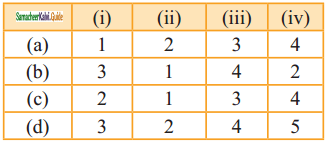b) 3 1 4 2

Question 22.
Consider the following reversible reaction at equilibrium, A + B ⇌ C, If the concentration of the reactants A and B are doubled, then the equilibrium constant will
a) be doubled
b) become one fourth
c) be halved
d) remain the same
d) remain the same

Question 23.
[Co(H2O)6]2+ (aq) (pink) + 4Cl (aq) ⇌ [CoCl4]2-(aq) (blue) + 6 H2O (l)
In the above reaction at equilibrium, the reaction mixture is blue in colour at room temperature. On cooling this mixture, it becomes pink in color. On the basis of this information, which one of the following is true?
a) ∆H > 0 for the forward reaction
b) ∆H = 0 for the reverse reaction
c) ∆H < 0 for the forward reaction
d) Sign of the ∆H cannot be predicted based on this information
a) ∆H > 0 for the forward reaction

Question 24.
The equilibrium constants of the following reactions are:
N2 + 3H2 ⇌ 2NH3; K1
N2 + O2 ⇌ 2NO; K2
H2 + 1/2O2 ⇌ H2O; K3
The equilibrium constant (K) for the reaction;
2NH3 + 5/2 O2 ⇌ 2NO + 3H2O, will be
a) K23 K3/K1

b) K1 K33/K2

c) K2K33/K1

d) K2K3/K1
c) K2K33/K1

Question 25.
A 20 litre container at 400 K contains CO2 (g) at pressure 0.4 atm and an excess (neglect the volume of solid SrO). The volume of the container is now decreased by moving the movable piston fitted in the container. The maximum volume of the container, when the pressure of CO2 attains its maximum value will be: Given that: SrCO3(S) ≅ SrO + CO2 (g) [Kp = 1.6 atm]
a) 2 litre
b) 5 litre
c) 10 litre
d) 4 litre
b) 5 litreII. Write brief answer to the following questions:

Question 26.
If there is no change in concentration, why is the equilibrium state considered dynamic?
At chemical equilibrium the rate of two opposing reactions are equal and the concentration of. reactants and products do not change with time. This condition is not static and is dynamic because both the forward and reverse reactions are still occurring at the same rate and no macroscopic change is observed. So the chemical equilibrium is in a state of dynamic equilibrium.

Question 27.
For a given reaction at a particular temperature, the equilibrium constant has a constant value. Is the value of Q also constant? Explain.
Kc and Qc are constant at equilibrium, both are temperature dependent. When Kc is constant at a given temperature, Qc also constant.

Question 28.
What is the relation between Kp and Kc? Given one example for which Kp is equal to Kc.
The relation between Kp and Kc is Kp = KC (RT)∆ng
Kp = equilibrium constant is terms of partial pressure.
Kc = equilibrium constant is terms of concentration.
R = gas constant
T = Temperature.
∆ng = Difference between the sum of the number of moles of products and the sum of number of moles of reactants in the gas phase. When ∆ng = 0
Kp = KC(RT)0 = KC i.e., Kp = KC
Example: H2(g) + I2(g) $$\rightleftharpoons$$ 2HI(g)
∆ng = 2 – 2 = 0
∴ Kp = KC for the synthesis of HI.

Question 29.
For a gaseous homogeneous reaction at equilibrium, number of moles of products are greater than the number of moles of reactants. Is Kc is larger or smaller than Kp.
Kp >Kc
np > nR

Question 30.
When the numerical value of the reaction quotient (Q) is greater than the equilibrium constant, in which direction does the reaction proceed to reach equilibrium?
When Q > KC the reaction will proceed in the reverse direction, i.e, formation of reactants.Question 31.
For the reaction, A2(g) + B2(g) ⇌ 2AB(g); ∆H is -ve.
the following molecular scenes represent differenr reaction mixture.(A-green, B-blue)i) Calculate the equilibrium constant Kp and (Kc).
ii) For the reaction mixture represented by scene (x), (y) the reaction proceed in which directions?
iii) What is the effect of increase in pressure for the mixture at equilibrium.
Kc = $$\frac{[\mathrm{AB}]^{2}}{\left[\mathrm{~A}_{2}\right]\left[\mathrm{B}_{2}\right]}$$ ; A – green, B – blue
Given that ‘V’ is constant(closed system)
At equilbrium,
Kc = $$\frac{(-)}{\left(\frac{2}{V}\right)\left(\frac{2}{V}\right)}$$ = $$\frac{16}{4}$$ = 4
Kp = Kc(RT)∆n = 4 (RT)0 = 4
At stage ‘x’,
Q = $$\frac{\left(\frac{6}{\mathrm{~V}}\right)^{2}}{\left(\frac{2}{\mathrm{~V}}\right)\left(\frac{1}{\mathrm{~V}}\right)}=\frac{36}{2}$$ = 18
Q > Kc i.e., reverse reaction is favoured.
At stage ‘y’,
Q = $$\frac{\left(\frac{3}{V}\right)^{2}}{\left(\frac{3}{V}\right)\left(\frac{3}{V}\right)}=\frac{9}{3 \times 3}$$ = 1
Kc > Q i.e., forward reaction is favoured.

Question 32.
State Le – Chateller principle.
It states that If a system at equilibrium is disturbed, then the system shifts itself in a direction that nullifies the effect of that disturbance.

Question 33.
Consider the following reactions,
a) H2(g) + I2(g) ⇌ 2HI(g)
b) CaCO3(s) ⇌ CaO (s) + CO2(g)
c) S(s) + 3 F2(g) ⇌ SF6(g)
In each of the above reactions find out whether you have to increase (or) decrease the volume to increase the yield of the product.
a) H2(g) + I2(g) ⇌ 2HI(g)
In this reaction, there is no effect on changing the volume. ∆ng = 0

b) CaCO3(s) ⇌ CaO (s) + CO2(g)
In this reaction, increases in volume favours forward reaction.

c) S(s) + 3 F2(g) ⇌ SF6(g)
In this reaction, decreases in volume favours forward reaction.

Question 34.
State law of mass action.
The law states that “At any instant, the rate of chemical reaction at a given temperature is directly proportional to the product of the active masses of the reactants at that instant.”Question 35.
Explain how will you predict the direction of an equilibrium reaction.
From the knowledge of equilibrium constant, it is possible to predict the di¬rection in which the net reaction is taking place for a given concentration or partial pressure of reactants and products.
Consider a general homogeneous reversible reaction,

xA + yB ⇌ lC + mD

For the above reaction under non-equilibrium conditions, reaction quotient ‘Q’ is defined as the ratio of the product of active masses of reaction products raised to the respective stoichiometric coefficients in the balanced chemical equation to that of the reactants.

Under non – equilibrium conditions, the reaction quotient Q can be calculated using the following expression.
Q = $$\frac{[\mathrm{C}]^{1}[\mathrm{D}]^{\mathrm{m}}}{[\mathrm{A}]^{\mathrm{x}}[\mathrm{B}]^{\mathrm{y}}}$$

As the reaction proceeds, there is a continuous change in the concentration of reactants and products and also the Q value until the reaction reaches equilibrium. At equilibrium, Q is equal to Kc at a particular temperature. Once the equilibrium is attained, there is no change in the Q value. By knowing the Q value, we can predict the direction of the reaction by comparing it with Kc.
If Q = Kc, the reaction is in equilibrium state.
If Q > Kc, the reaction will proceed in the reverse direction, i.e., formation of reactants.
If Q < Kc, the reaction will proceed in the forward direction i.e., formation of products.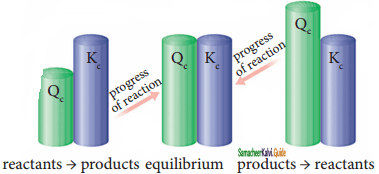Predicting the direction of a reaction

Question 36.
Derive a general expression for the equilibrium constant Kp and Kc for the reaction, 3H2(g) + N2(g) ⇌ 2NH3(g).
Let us consider the formation of ammonia in which, ‘a’ moles nitrogen and h’ moles hydrogen gas are allowed to react in a container of volume V. Let ‘x’ moles of nitrogen react with 3 x moles of hydrogen to give 2x moles of ammonia.
3H2(g) + N2(g) ⇌ 2NH3(g)Applying law of mass action,
K2 = $$\frac{\left[\mathrm{NH}_{3}\right]^{2}}{\left[\mathrm{~N}_{2}\right]\left[\mathrm{H}_{2}\right]^{3}}$$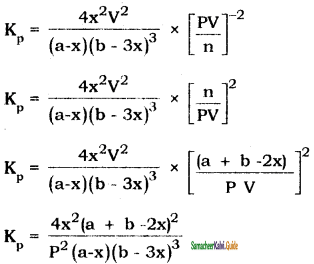Question 37.
Write the balanced chemical equation for an equilibrium reaction for which the equilibrium constant is given by expression.
Kc = $$\frac{\left[\mathrm{NH}_{3}\right]^{4}\left[\mathrm{O}_{2}\right]^{7}}{\left.[\mathrm{NO}]^{4} \mid \mathrm{H}_{2} \mathrm{O}\right]^{6}}$$
Balanced equation is:
4NO + 6H2O ⇌ 4NH3 + 7O2

Question 38.
What is the effect of added Inert gas on the reaction at equilibrium?
When an inert gas (i.e., a gas which does not react with any other species involved in equilibrium) is added to an equilibrium system at constant volume, the total number of moles. of gases present in the container increases, that is, the total pressure of gases increases, the partial pressure of the reactants and the products are unchanged. Hence at constant volume, the addition of inert gas has no effect on the equilibrium.Question 39.
Derive the relation between Kp and Kc.
Let us consider the general reaction in which all reactants and products are ideal gases.
xA + yB ⇌ lC + mD
The equilibrium constant, Kc is,
Kc = $$\frac{\left[\mathrm{Cl}^{l}[\mathrm{D}]^{\mathrm{m}}\right.}{[\mathrm{A}]^{\mathrm{x}}[\mathrm{B}]^{\mathrm{y}}}$$ ……………..(1)

and Kp is,
Kp = $$\frac{p_{C}^{l} \times p_{D}^{m}}{p_{A}^{x} \times p_{B}^{y}}$$ ………….(2)

The ideal gas equation is,
PV = nRT
P = $$\frac{n}{V}$$ RT
since, Active mass = molar concentration = n/V
P = active mass × RT
Based on the above expression the partial pressure of the reactants and products can be expresssed as,
PXA = [A]x[RT]x
PYB = [A]y[RT]y
PlC = [A]l[RT]l
PmD = [A]m[RT]m
On substituting in Eqn.(2).,By comparing equation (1) and (4),
we get
Kp∆ng =Kc (RT) …………..(5)
Where,
∆ng is the difference between the sum of number of moles of products and the sum of number of moles of reactants in the gas phase.
The following relations become immediately obvious.
when ∆ng = 0.
Kp = Kc (RT)0 = Kc
Example :
H2(g) + I2(g) ⇌ 2HI(g)
N2 + O2 ⇌ 2NO(g)
when ∆ng = +ve
Kp = Kc(RT)+ve
Kp > Kc
Example:
2NH3(g) ⇌ N2(g) + 3H2(g)
PCl5(g) ⇌ PCl3(g) + Cl2(g)
When ∆ng = -ve
Kp = Kc(RT)-ve
Kp < Kc
Example:
2H2(g) + O2(g) ⇌ 2H2O(g)
2SO2(g)+ O2(g) ⇌ 2SO3(g)

Question 40.
One mole of PCl5 is heated in one litre closed container. If 0.6 mole of chlorine is found at equilibrium, Calculate the value of equilibrium constant.
Solution:
Given that,
[PCl5]initial = $$\frac{1 \text { mole }}{1 \mathrm{dm}^{3}}$$
[Cl2]eq = 0.6 mole dm-3
PCl5 ⇌ PCl3 + Cl2
[PCl3]eq = 0.6 mole dm-3
[PCl5]eq = 0.4 mole dm-3
∴ Kc = $$\frac{\left[\mathrm{PCl}_{3}\right]\left[\mathrm{Cl}_{2}\right]}{\left[\mathrm{PCl}_{5}\right]}=\frac{0.6 \times 0.6}{0.4}$$
= 0.9 mole dm-3

Question 41.
For the reaction SrCO3 (s) ⇌ SrO(s) + CO2(g) the value of equilibrium constant Kp = 2.2 × 10-4 at 1002 K. Calculate Kc for the reaction.
Solution :
For the reaction,
SrCO3(s) ⇌ SrO(s) + CO2(g)
∆ng = 1 – 0 = 1
∴ Kp = Kc (RT)
2.2 × 10-4 = Kc (0.0821) (1002)
Kc = $$\frac{2.2 \times 10^{-4}}{0.0821 \times 1002}$$ = 2.674 × 10-6

Question 42.
To study the decomposition of hydrogen iodide, a student fills an evacuated 3 litre flask with 0.3 mol of HI gas and allows the reaction to proceed at 500°C. At equilibrium he found the concentration of HI which is equal to 0.05 M. Calculate Kc and Kp.
Solution :
V = 3 L
[HI]initial = $$\frac{0.3 \mathrm{~mol}}{3 \mathrm{~L}}$$ 0.1 M
[HI]eq = 0.05 M
2 HI(g) ⇌ H2(g) + I2(g)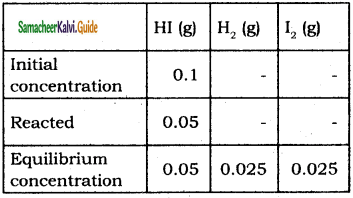Kc = $$\frac{\left[\mathrm{H}_{2}\right]\left[\mathrm{I}_{2}\right]}{[\mathrm{HI}]^{2}}=\frac{0.025 \times 0.025}{0.05 \times 0.05}$$ = 0.25
Kp = Kc(RT)(∆ng)
∆ng = 2 – 2 = 0
Kp = 0.25(RT)0
Kp = 0.25.Question 43.
Oxidation of nitrogen monoxide was studied at 200 with initial pressures of 1 atm NO and 1 atm of O2. At equilibrium partial pressure of oxygen is found to be 0. 5 atm calculate Kp value.
Solution:
2 NO(g) + O2(g) ⇌ 2NO2(g)Kp = $$\frac{\left(P_{N O_{2}}\right)^{2}}{\left(P_{N O}\right)^{2}\left(P_{O}\right)}$$

= $$\frac{0.96 \times 0.96}{0.04 \times 0.04 \times 0.52}$$
Kp = 1.017 × 103.

Question 44.
1 mol of CH4, 1 mole of CS2 and 2 mol of H2S are 2 mol of H2 are mixed in a 500 ml flask. The equilibrium constant for the reaction Kc = 4 x 10-2 mol2 lit-2. In which direction will the reaction proceed to reach equilibrium ?
Solution :
CH4(g) + 2 H2S (g) ⇌ CS2(g) + 4H2(g)
Kc = 4 x 10-2 mol2 lit-2
Volume = 500 ml = $$\frac{1}{2}$$ L
[CH4]in = $$\frac{1 \mathrm{~mol}}{\frac{1}{2} L}$$ = 2 mol L-1;

[CH2]in = $$\frac{1 \mathrm{~mol}}{\frac{1}{2} L}$$ = 2 mol L-1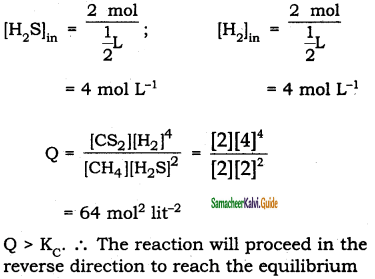Question 45.
At particular temperature Kc = 4 × 10-2 for the reaction, H2S ⇌ 2H2(g) + S2(g). Calculate the Kc for each of the following reaction.
i) 2H2S(g) ⇌ 2H2 + S2(g)
ii) 3H2S(g) ⇌ 3H2(g) + 3/2 S2(g)
Solution :
Kc = 4 × 10-2 for the reaction,
H2S ⇌ 2H2(g) + S2(g)
Kc = $$\frac{\left[\mathrm{H}_{2}\right]\left[\mathrm{S}_{2}\right]^{1 / 2}}{\left[\mathrm{H}_{2} \mathrm{~S}\right]}$$

⇒ 4 × 10-2 = $$\frac{\left[\mathrm{H}_{2}\right]\left[\mathrm{S}_{2}\right]^{1 / 2}}{\left[\mathrm{H}_{2} \mathrm{~S}\right]}$$

(i) 2H2S(g) ⇌ 2H2 + S2(g)
For the reaction,
2H2S(g) ⇌ 2H2 + S2(g)
Kc = $$\frac{\left[\mathrm{H}_{2}\right]^{2}\left[\mathrm{~S}_{2}\right]}{\left[\mathrm{H}_{2} \mathrm{~S}\right]^{2}}$$
= (4 × 10-2)2 = 16 × 10-4

(ii) 3H2S(g) ⇌ 3H2(g) + 3/2 S2(g)
For the reaction,
3H2S(g) ⇌ 3H2(g) + 3/2 S2(g)
Kc = $$\frac{\left[\mathrm{H}_{2}\right]^{3}\left[\mathrm{~S}_{2}\right]^{3 / 2}}{\left[\mathrm{H}_{2} \mathrm{~S}\right]^{3}}$$
= (4 × 10-2)3 = 64 × 10-6Question 46.
28 g of Nitrogen and 6 g of hydrogen were mIed In a 1 litre closed container. At equIlibrium 17 g NH3 was produced. Calculate the weight of nitrogen, hydrogen at equilibrium.
Solution:
Given mN2 =28g;
mH2 = 6g;
V = 1 L.
(nN2)initial = $$\frac{28}{28}$$ = 1

(nH2)initial = $$\frac{6}{2}$$ = 3
N2(g) + 3 H2(g) ⇌ 2NH3(g)[NH3] = $$\frac{17}{17}$$ = 1 mol
Weight of N2 = (no. of moles of N2) × (molar mass of N2)
= 0.5 × 28 = 14g
Weight of H2 = (no. of moles of H2) × (molar mass of H2)
= 1.5 × 2 = 3g

Question 47.
The equilibrium for the dissociation of XY2 is given as,
2 XY2(g) ⇌ 2 XY(g) + Y2(g)
if the degree of dissociation x is so small compared to one. Show that 2 Kp = PX3 where P is the total pressure and Kp is the dissociation equilibrium constant of XY2.
2 XY2(g) ⇌ 2 XY(g) + Y2(g)Question 48.
A sealed container was filled with 0.3 mol H2(g), 0.4 mol I2(g) and 0.2 mol HI(g) at 800 K and total pressure 1.00 bar. Calculate the amounts of the components in the mixture at equilibrium given that K = 870 for the reaction, H2(g) + I2(g) ⇌ 2 HI (g).
Solution:
A2(g) + B2(g) ⇌ 2 AB (g)Given that, Kp = 1;
$$\frac{4 x^{2}}{(1-x)^{2}}$$ = 1
⇒ 4x2 = (1 – x)2 = 1
⇒ 4x2 = 1 + x2 – 2x
3x2 + 2x – 1 = 0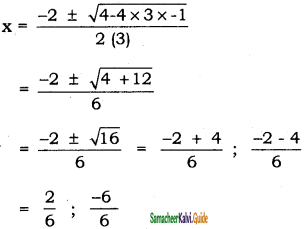x = 0.33 – 1(not possible)
∴ [A2]eq = 1 – x = 1 – 0.33 = 0.67
[B2]eq = 1 – x = 1 – 0.33 = 0.67
[AB2]eq = 2x × 0.33 = 0.66

Question 49.
Deduce the Vant Hoff equation.
This equation gives that quantitative temperature dependence of equilibrium constant (K). The relation between standard free energy change (∆G°) and equilibrium constant is
∆G° = – RT In K ………………..(1)
We know that, ∆G° = ∆H° – T∆S° …………(2)
Substituting (1) in equation (2)
– RT In K = ∆H° – T∆S°
Rearranging, In K = $$\frac{\Delta \mathrm{H}^{0}}{\mathrm{RT}}+\frac{\Delta \mathrm{S}^{0}}{\mathrm{RT}}$$ ……………(3)
Differentiating equation (3) with respect to temperature,
$$\frac{\mathrm{d}(\ln \mathrm{K})}{\mathrm{dT}}=\frac{\Delta \mathrm{H}^{0}}{\mathrm{RT}^{2}}$$ ……………….(4)
Equation (4) is known as differential form of van,t Hoff equation.
On integrating the equation (4), between T1 and T2 with their respective equilbrium consatnts K1 and K2.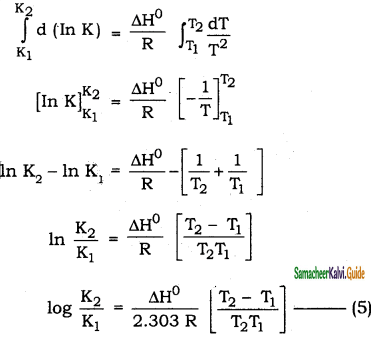Equation 5 is known as integrated form of Van’t Hoff equation.

Question 50.
The equilibrium constant Kp for the reaction N2(g) + 3H2(g) ⇌ 2NH3(g) is 8.19 × 102 at 298 K and 4.6 × 10-1 at 498 K. Calculate ∆H° for the reaction.
Solution :
Kp1 = 8.19 × 102;
T1 = 298 K
Kp1 = 8.19 × 102;
T1 = 298 K
Kp2 = 4.16 × 10-1;
T2 = 498 K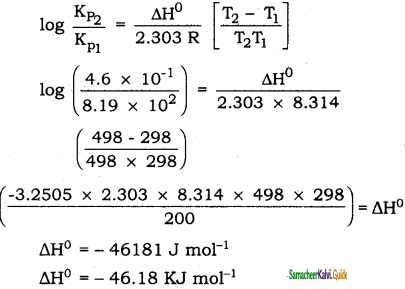Question 51.
The partial pressure of carbon dioxide in the reaction CaCO3(s) ⇌ CaO(s) + CO2(g) is 1.017 × 10-3 atm at 500°C. Calculate Kp at 600°C for the reaction. H for the reaction is 181 KJ mol-1 and does not change in the given range of temperature.
Solution:
PCO2 = 1.017 × 10-3 atm
T = 500°C;
Kp = PCO2
∴ Kp1 = 1.017 × 10-3;
T = 500 + 273 = 773 K
Kp2 = ?
T = 600 + 273 = 873 K
∆H° = 181 KJ mol-1### 11th Chemistry Guide Physical and Chemical Equilibrium Additional Questions and Answers

Question 1.
Which of the following represents physical equilibrium?
(a) PCl5(g) $$\rightleftharpoons$$ PCl3(g) + Cl2(g)
(b) H2(g) + I2(g) $$\rightleftharpoons$$ 2HI(g)
(c) H2O(l) $$\rightleftharpoons$$ H2O(g)
(d) N2(g) + 3H2(g) $$\rightleftharpoons$$ 2NH3(g)
(c) H2O(l) $$\rightleftharpoons$$ H2O(g)
Solution:
Physical states arc in equilibrium i.e., liquid – vapour equilibrium.

Question 2.
In which of the following reaction, the value of Kp will be equal to Kc?
a) H2 + I2 ⇌ 2HI
b) PCl5 ⇌ PCl3 + Cl2
c) 2NH3 ⇌ N2 + 3H2
d) 2SO2 +O2 ⇌ 2SO3
a) H2 + I2 ⇌ 2HI

Question 3.
Which one of the following does not undergo sublimation?
(a) Iodine
(b) water
(c) Camphor
(d) Ammonium chloride
(b) Water

Question 4.
The reaction, 2SO2(g) + O2(g) ⇌ 2SO3(g) is carried out in a 1 dm3 vessel and 2 dm3 vessels separately. The ratio of the reaction velocities will be
a) 1 : 8
b) 1 : 4
c) 4 : 1
d) 8 : 1
d) 8 : 1

Question 5.
Which of the following is an example of homogeneous equilibrium?
(a) H2O(1) $$\rightleftharpoons$$ H2O(g)
(b) CaCO3(s) $$\rightleftharpoons$$ CaO(s) + CO(g)
(c) H2(g) + I2(g) $$\rightleftharpoons$$ 2HI(g)
(d) 2CO(g) $$\rightleftharpoons$$ CO2(g) + C(s)
(c) H2(g) + I2(g) $$\rightleftharpoons$$ 2HI(g)
Solution:
Here all reactants and products are in the same phase i.e., gaseous phase.Question 6.
The equilibrium constant expression for the equilibrium :
2NH2(g) + 2O2(g) ⇌ N2O(g) + 3H2O(g) is
a) Kc = $$\frac{\left[\mathrm{N}_{2} \mathrm{O}\right]\left[\mathrm{H}_{2} \mathrm{O}\right]^{3}}{\left[\mathrm{NH}_{3}\right]\left[\mathrm{O}_{2}\right]}$$

b) Kc = $$\frac{\left[\mathrm{H}_{2} \mathrm{O}\right]^{3}\left[\mathrm{~N}_{2} \mathrm{O}\right]}{\left[\mathrm{NH}_{3}\right]^{2}\left[\mathrm{O}_{2}\right]^{2}}$$

c) Kc = $$\frac{\left[\mathrm{NH}_{3}\right]^{2}\left[\mathrm{O}_{2}\right]^{2}}{\left[\mathrm{~N}_{2} \mathrm{O}\right]\left[\mathrm{H}_{2} \mathrm{O}\right]^{3}}$$

d) Kc = $$\frac{\left[\mathrm{NH}_{3}\right]\left[\mathrm{O}_{2}\right]}{\left[\mathrm{N}_{2} \mathrm{O}\right]\left[\mathrm{H}_{2} \mathrm{O}\right]}$$
b) Kc = $$\frac{\left[\mathrm{H}_{2} \mathrm{O}\right]^{3}\left[\mathrm{~N}_{2} \mathrm{O}\right]}{\left[\mathrm{NH}_{3}\right]^{2}\left[\mathrm{O}_{2}\right]^{2}}$$

Question 7.
Statement I: In dissociation of PCI5 to PCI3 and CI2, Kp > KC
Statement II: In dissociation of PCI5, Δng = – ve and so Kp > KC.
(a) Statement I & II are correct and statement II is the correct explanation of statement I.
(b) Statement I & II are correct but statement II is not the correct explanation of statement I.
(c) Statement I is correct but statement II is wrong.
(d) Statement I is wrong but statement II is correct.
(c) Statement I is correct but statement II is wrong.
Solution:
Δng = 2 – 1 = 1 = +ve

Question 8.
For the reaction PCl3(g) + Cl2(g) ⇌ PCl5(g) at 250°C, the value of Kc is 26, then the value of Kp at the same temperature will be
a) 0.61
b) 0.57
c) 0.83
d) 0.46
a) 0.61

Question 9.
In which of the following reaction, Kp is equal to KC ?
(a) N2(g) ± O2(g) $$\rightleftharpoons$$ 2NO(g)
(b) 2NH3(g) $$\rightleftharpoons$$ N2(g) + 3H2(g)
(c) 2H2(g) + O2(g) $$\rightleftharpoons$$ 2H2O(g)
(d) PCI5(g) $$\rightleftharpoons$$ PCI3(g) + CI2
(a) N2(g) ± O2(g) $$\rightleftharpoons$$ 2NO(g)
Solution:
KC (RT)Δng when Δng = 0 then K = K for option (a), Δng = 2 – 2 = 0

Question 10.
In which of the following equilibria, the value of Kp is less than Kc?
a) H2 + I2 ⇌ 2HI
b) N2 + 3 H2 ⇌ 2NH3
c) N2 + O2 ⇌ 2NO
d) CO + H2O ⇌ CO2 + H2
b) N2 + 3 H2 ⇌ 2NH3Question 11.
In the reversible reaction A + B ⇌ C + D. The concentration of each C and D at equilibrium was 0.8-mole lit, then the equilibrium constant will be
a) 6.4
b) 0.64
c) 1.6
d) 16.0
d) 16.0

Question 12.
Statement I: A pure solid in an equilibrium reaction has the same concentration at a given temperature.
Statement II: The solid does not expand to fill its container and it has the same number of moles of its volume.
(a) Statement I and II are correct and statement II is the correct explanation of the statement of I.
(b) Statement I and II are correct but II is not the correct explanation of!.
(c) Statement I and II are not correct.
(d) Statement I is wrong but 11 is correct.
(a) Statement I and II are correct and statement II is the correct explanation of the statement of I.

Question 13.
For the reaction C(s) + CO2(g) ⇌ 2CO (g), the partial pressure of CO2 and CO are 2.0 and 4.0 atm respectively at equilibrium. The Kp for the reaction is
a) 0.5
b) 4.0
c) 8.0
d) 32.0
c) 8.0

Question 14.
When the rate of forward reaction becomes equal to backward reaction, this state is termed as
a) chemical equilibrium
b) Reversible state
c) Equilibrium
d) All of these
d) All of these

Question 15.
Which of the following does not alter the equilibrium?
(a) catalyst
(b) concentration
(c) temperature
(d) pressure
(a) catalystQuestion 16.
For the reaction PCl3(g) + Cl2(g) ⇌ PCl5(g), the position of equilibrium can be
shifted to the right by _______.
a) increasing the temperature
b) Doubling the volume
c) Addition of Cl2 at constant volume
d) Addition of equimolar quantities of PCl3 and PCl5
b) Doubling the volume

Question 17.
In which of the following reaction, pressure has no effect?
(a) N2 + 3N2 $$\rightleftharpoons$$ 2NH3(g)
(b) 2SO2(g) + O2(g) $$\rightleftharpoons$$ 2SO3(g)
(c) N2O4(g) $$\rightleftharpoons$$ 2NO2(g)
(d) H2(g) + I2(g) $$\rightleftharpoons$$ 2HI(g)
(d) H2(g) + I2(g) $$\rightleftharpoons$$ 2HI(g)
Solution:
In the reaction H2(g) + I2(g) $$\rightleftharpoons$$ 2HI(g) the volumes are equal on both sides and so pressure has no effect.

Question 18.
Rate of reaction curve for equilibrium can be like [rf = forward rate, rb = backward rate]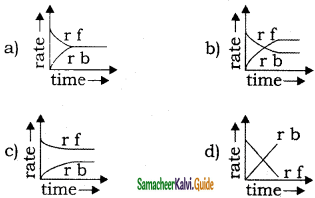a)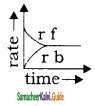Question 19.
For a reaction, N2 + 3H2 ⇌ 2NH3(g), the value of Kc does not depend upon
A) initial concentration of the reactants
B) pressure
C) temperature
D) Catalyst
a) only C
b) A, B, C
c) A, B, C
d) A, B, C, D
c) A, B, C

Question 20.
Which one of the following equations is not correct?
(a) ΔG° = – RTInK
(b) ΔG° = ΔH° – TΔS°
(c) – RTInK = ΔH° – TΔS°
(d) In k = $$\frac { ΔH° }{ T }$$ – $$\frac { ΔS° }{ R }$$
(d) In k = $$\frac { ΔH° }{ T }$$ – $$\frac { ΔS° }{ R }$$Question 21.
At 1000 K, the value of Kp for the reaction, A(g) + 2B(g) ⇌ 3C (g) + D(g) is 0.05 atm. The value of Kc in terms of R would be
a) 20000 R
b) 0.02 R
c) 5 × 10-5 R
d) 5 × 10-5 × R-1
d) 5 × 10-5 × R-1

Question 22.
In a chemical equilibrium, the rate constant for the backward reaction is 7.5 x 10-4 and the equilibrium constant is 1.5. The rate constant for the forward reaction
a) 2 × 10-3
b) 5 × 10-4
c) 1.12 × 10-3
d) 9.0 × 10-4
c) 1.12 × 10-3

Question 23.
A reversible reaction is one which
a) Proceeds in one direction
b) proceeds in both direction
c) proceeds spontaneously
d) All the statements are wrong
b) proceeds in both direction

Question 24.
What is the correct expression for the representation of the solubility product constant of Ag2CrO4?
(a) [Ag+]2 [CrO42-]
(b) [2Ag+] [CrO42-]
(c) [Ag+] [CrO42-]
(d) [2Ag+]2 [CrO42-]
(a) [Ag+]2 [CrO42-]

Question 25.
For the system A(g) + 2B(g) ⇌C (g), the equilibrium concentrations are (A) 0.06 mole / lit (B) 0.12 mole / lit and (C) 0.216 mole / lit. The Keq for the reaction is
a) 250
b) 416
c) 4 × 10-3
d) 125
a) 250Question 26.
What effect does a catalyst have on the equilibrium position of a reaction?
(a) a catalyst favours the formation of products
(b) a catalyst favours the formation of reactants
(c) a catalyst does not change the equilibrium position of a reaction
(d) a catalyst may favour reactants or product formation, depending upon the direction in which the reaction is written.
(c) a catalyst does not change the equilibrium position of a reaction

Question 27.
Partial pressures of A, B, C and D on the basis of gaseous system A + 2B ⇌ C + 3D are A = 0.20, B = 0.10, C = 0.30 and D = 0.50 atm. The numerical value of equilibrium constant is _______.
a) 11.25
b) 18.75
c) 5
d) 3.75
b) 18.75

Question 28.
The molar concentration of 96 g of O2 contained in a 2 L vessel is ______.
a) 16 mol / L
b) 1.5 mol / L
c) 4 mol / L
d) 24 mol / L
b) 1.5 mol / L

Question 29.
According to the law of mass action rate of a chemical reaction is proportional to
a) concentration of reactants
b) molar concentration of reactants
c) concentration of products
d) molar concentration of products
b) molar concentration of reactants

Question 30.
The equilibrium constant of the reaction SO2(g) + $$\frac{1}{2}$$ O2(g) ⇌ SO3 (g) is 4 × 10-3 atm-1/2. The equilibrium constant of the reaction 2SO3(g) ⇌ 2SO2(g) + O2(g) would be
a) 250 atm
b) 4 × 103 atm
c) 0.25 × 104 atm
d) 6.25 × 104 atm
d) 6.25 × 104 atmQuestion 31.
Which of the following is not a general characteristic of equilibria involving physical processes?
(a) Equilibrium is possible only in a close system at a given temperature
(b) All measurable properties of the system remain constant
(c) All the physical processes stop at equilibrium
(d) The opposing processes occur at the same rate and there is a dynamic but stable condition
(c) All the physical processes stop at equilibrium

Question 32.
The active mass of 64 g of HI is a two-liter flask would be
a) 2
b) 1
c) 5
d) 0.25
d) 0.25

Question 33.
At a certain temperature, 2HI ⇌ H2 + I2. Only 50 % HI is dissociated at equilibrium. The equilibrium constant is
a) 0.25
b) 1.0
c) 3.0
d) 0.50
a) 0.25

Question 34.
Unit of equilibrium constant for the reversible reaction, H2 + I2 ⇌ 2HI is ________.
a) mol-1 litre
b) mol-2 litre
c) mol litre-1
d) None of these
d) None of these

Question 35.
Consider the reaction CaCO3(s) $$\rightleftharpoons$$ CaO(s) + CO2(g) is a closed container at equilibrium. What would be the effect of the addition of CaCO3 on the equilibrium?
(a) increases
(b) remains unaffected
(c) decreases
(d) unpredictable
(b) remains unaffectedQuestion 36.
The following equilibrium are given
N2 +3H2 ⇌ 2NH2 ………K1
N2 + O2 ⇌ 2N0 ………….K2
H2 + 1/2 O2 ⇌ H2O …….. K3
The equilibrium constant of the reaction.
2 NH3 + 5/2 O2 ⇌ 2NO + 3H2O, in terms of K1, K2 and K3 is _________.
a) $$\frac{\mathbf{K}_{1} \mathbf{K}_{2}}{\mathbf{K}_{3}}$$

b) $$\frac{\mathrm{K}_{1} \mathrm{~K}_{3}^{2}}{\mathrm{~K}_{2}}$$

c) $$\frac{\mathrm{K}_{2} \mathrm{~K}_{3}^{2}}{\mathrm{~K}_{1}}$$

d) K1 K2 K3
c) $$\frac{\mathrm{K}_{2} \mathrm{~K}_{3}^{2}}{\mathrm{~K}_{1}}$$

Question 37.
For the reaction
2NO2(g) ⇌ 2NO(g) + O2(g) Kc = 1.8 × 10-6 at 184°C and R = 0.083 Jk-1 mol-1. When Kp and are compared at 184C, it
is found that
a) Kp > Kc
b) Kp < Kc
c) Kp – Kc
d) Kp × Kc( depends upon pressure of gases)
a) Kp > Kc

Question 38.
The rate of forwarding reaction is two times that of reverse reaction at a given temperature and identical concentration. K equilibrium is
a) 2.5
b) 2.0
c) 0.5
d) 1.5
b) 2.0

Question 39.
Hemoglobin (Hb) forms a bond with oxygen and given oxyhemoglobin (HbO2). This process is partially regulated by the concentration of H3O+ and dissolved CO2 in blood as HbO2 +H3O+ + CO2 $$\rightleftharpoons$$ H+ – Hb – CO2 + O2 + H2O. If there is the production of lactic acid and CO2 during a muscular exercise, then
(a) more HbO2 is formed
(b) more O2 is released
(c) CO2 is released
(d) both (b) and (c)
(b) more O2 is released

Question 40.
For the following thre. reactions 1, 2 and 3 equilibrium constants are given:
1. CO(g) + H2O(g) ⇌ CO(g) + H2(g); K1
2.CH4(g) + H2O(g) ⇌ CO + 3H(g); K2
3. CH4(g) + 2H2O(g) ⇌ CO(g) + 4H2(g); K3
Which of the following relations is correct?
a) K1√K2 = K3
b) K2K3 = K1
c) K3 = K1K2
d) K3 = K23 K12
c) K3 = K1K2Question 41.
4 moles of A is mixed with 4 moles of B. At equilibrium for the reaction A + B ⇌ C + D. 2 moles of C and D is formed. The equilibrium constant for the reaction will be
a) $$\frac{1}{4}$$
b) $$\frac{1}{2}$$
c) 1
d) 4
c) 1

Question 42.
In a reaction PCl5 ⇌ PCl3 + Cl2 degree of dissociation is 30%. If the Initial moles of PCl5 is one then total moles at equilibrium is
a) 1.3
b) 0.7
c) 1.6
d) 1.0
a) 1.3

Question 43.
When 3 moles of A and 1 mole of B are mixed in a 1 lit vessel, the following reaction takes place A(g) + B(g) ⇌ 2C(g), 1.5 moles of ‘C’ are formed. The equilibrium constant for the reaction is
a) 0.12
b) 0.25
c) 0.50
d) 4.0
d) 4.0

Question 44.
In which of the following, the reaction proceeds towards completion
a) K = 103
b) K = 10-2
c) K = 10
d) K = 1
a) K = 103

Question 45.
Two moles of NH3 when putting into a previously evacuated vessel (1L) partially dissociate into N2 and H2. If at equilibrium one mole of NH3 is present, the equilibrium constant is ________.
a) $$\frac{3}{4}$$ mol2 lit-2

b) $$\frac{27}{64}$$ mol2 lit-2

c) $$\frac{27}{32}$$ mol2 lit-2

d) $$\frac{27}{16}$$ mol2 lit-2
d) $$\frac{27}{16}$$ mol2 lit-2Question 46.
For the reaction N2(g) + O2(g) ⇌ 2NO(g) the value of Kc at 800°C is 0.1. When the equilibrium concentration of both reactions is 0.5 mol, what is the value of Kp at the same temperature?
a) 0.5
b) 0.1
c) 0.01
d) 0.025
b) 0.1

Question 47.
28 g of N2(g) and 6g of H2(g) were mixed at equilibrium 17 g NH3 was produced. The weight of N2 and H2 at equilibrium are respectively.
a) 11g, 0g
b) 1g, 3g
c) 14g, 3g
d) 11g, 3g
c) 14g, 3g

Question 48.
2SO3 ⇌ 2SO2 + O2 If Kc = 100, α = 1, half of the reaction is completed, the concentration of SO3 and SO2 are equal, the concentration of O2 is _________.
a) 0.001 M
b) $$\frac{1}{2}$$ SO2
c) 2 times of SO2
d) Data in complete
d) Data incomplete

Question 49.
For reaction HI ⇌ $$\frac{1}{2}$$H2 + $$\frac{1}{2}$$I2 value of Kc is $$\frac{1}{8}$$, then value of K is $$\frac{1}{8}$$ for H2 + I2 ⇌ 2HI.
a) $$\frac{1}{64}$$
b) 64
c) $$\frac{1}{8}$$
d) 8
b) 64

Question 50.
2 moles of PCl5 were heated in a closed vessel of 2-liter capacity. At equilibrium, 40% of PCl5 is dissociated into PCl3 and Cl2. The value of the equilibrium constant is
a) 0.266
b) 0.53
c) 2.66
d) 5.3
a) 0.266Question 51.
For a reaction H2 + I2 ⇌ 2HI at 721 k, the value of the equilibrium constant is 50. If 0.5 mol each of H2 and I2 is added to the system the value of the equilibrium constant will be
a) 40
b) 60
c) 50
d) 30
c) 50

Question 52.
In a reaction the rate of reaction is proportional to its active mass, this statement is known as
a) Law of mass action
b) Le – Chatelier principle
d) law of constant proportion
b) Le – Chatelier principle

Question 53.
In a chemical equilibrium A + B ⇌ C + D, when one mole each of the two reactants are mixed, 0.6 mole each of the products are formed. The equilibrium constant calculated is
a) 1
b) 0.36
c) 2.25
d) $$\frac{4}{9}$$
c) 2.25

Question 54.
Under a given set of experimental conditions, with an increase in the concentration of the reactants, the rate of a chemical reaction.
a) Decreases
b) Increases
c) Remains unaltered
d) First decreases and then increases
c) Remains unaltered

Question 55.
A + B ⇌ C + D, If finally the concentrations of A and B are both equal but at equilibrium concentration of D will be twice of that of A then what will be the equilibrium constant of reaction.
a) $$\frac{4}{9}$$
b) $$\frac{9}{4}$$
c) $$\frac{1}{9}$$
d) 4
d) 4Question 56.
Theory of ‘active mass’ indicates that the rate of chemical reaction is directly proportional to the ___________.
a) Equilibrium constant
b) Properties of reactants
c) Volume of apparatus
d) Concentration of reactants
d) Concentration of reactants

Question 57.
In the reaction, A + B ⇌ 2C, at equilibrium the concentration of A and B is 0.20 mol L-1 each and that of C was found to be 0.60 mol L-1. The equilibrium constant of the reaction is
a) 2.4
b) 18
c) 4.8
d) 9
d) 9

Question 58.
The rate at which substances react depends on their _______.
a) Atomic weight
b) Molecular weight
c) Equivalent weight
d) Active mass
c) Equivalent weight

Question 59.
If in the reaction N2O4 ⇌ 2NO2, α is that part of N2O4 which dissociates, then the number of moles at equilibrium will be
a) 3
b) 1
c) (1- α)2
d) (1 + α)
d) (1 + α)

Question 60.
On decomposition of NH4HS, the following equilibrium is established NH4HS(s) ⇌ NH3(g) + H2S(g). If the total pressure is P atm, then the equilibrium constant Kp is equal to _______.
a) P atm
b) P2 atm2
c) $$\frac{\mathrm{P}^{2}}{4}$$ atm2
d) 2P atm
c) $$\frac{\mathrm{P}^{2}}{4}$$ atm2Question 61.
Some gaseous equilibrium are given below:
i) CO + H2O ⇌ CO2 + H2
ii) 2CO + O2 ⇌ 2CO
iii) 2H2 + O2 ⇌ 2H3O
find out the relation between equilibrium constants.
a) K = K1K2
b) K = (K1K2)2
c) K = (K1K2)–$$\frac{1}{2}$$
d) K = $$\left(\frac{\mathrm{K}_{1}}{\mathrm{~K}_{2}}\right)^{\frac{1}{2}}$$
d) K = $$\left(\frac{\mathrm{K}_{1}}{\mathrm{~K}_{2}}\right)^{\frac{1}{2}}$$

Question 62.
Kc = 9 for the reaction A + B ⇌ C + D. If A and B are taken In equal amounts, the the amount of ‘C’ in equilibrium is
a) 1
b) 0.25
c) 0.75
d) None of these
c) 0.75

Question 63.
In the reaction C(S) + CO2(g) ⇌ 2CO(g) the equilibrium pressure is 12 atm. If 50 % of CO2 reacts, then Kp will be ________.
a) 12 atm
b) 16 atm
c) 20 atm
d) 24 atm
b) 16 atm

Question 64.
For the following gas equilibrium, N2O4(g) ⇌ 2NO2(g) Kp is found to be equal to Kc. This is attained when,
a) 0°C
b) 273 k
c) 1 k
d) 12.19 k
d) 12.19 k

Question 65.
Consider the following reversible gaseous reactions {At 298 K)
a) N2O4 ⇌ 2NO2
b) 2SO2 + O2 ⇌ 2SO3
c) 2HI ⇌ H2 + I2
d) X + Y ⇌ 4Z
a) N2O4 ⇌ 2NO2Question 66.
For the reaction A + 2B ⇌ 2C at equilibrium [C] = 1.4 M, [A]0 = 1 M, [B]0 = 2M, [C]0 = 3 M. The value of Kc is .
a) 0.084
b) 8.4
c) 84
d) 840
a) 0.084

Question 67.
For the reaction H2(g) + I2(g) ⇌ 2HI(g). Kc = 66.9 at 350°C and Kc = 50.0 at 448°C. The reaction has
a) ∆H = +ve
b) ∆H = -ve
c) ∆H = zero
b) ∆H = -ve

Question 68.
In an equilibrium reaction H2(g) + I2(g) ⇌ 2HI(g), ∆H = -3000 calories, which factor favours dissociation of HI
a) Low temperature
b) High pressure
c) High temperature
d) Low pressure
c) High temperature

Question 69.
In an equilibrium reaction for which ∆G°= 0, the equilibrium constant K Is
a) 0
b) 1
c) 2
d) 10
b) 1

Question 70.
Consider the following reversible reaction at equilibrium, 2H2O(g) ⇌ 2H2(g) + O2(g). Which one of the following ∆H = 241. 7 kJ changes in a conditions will lead to maximum decomposition of H2O(g)?
a) Increasing both temperature and pressure
b) Decreasing temperature and increasing pressure
c) Increasing temperature and decreasing pressure
d) Increasing temperature at constant pressure.
c) Increasing temperature and decreasing pressureQuestion 71.
In which of the following system doubling the volume of the container causes a shift to the right.
a) H2(g) + Cl2(g) ⇌ 2HCl(g)
b) 2CO(g) + O2(g) ⇌ 2CO2(g)
c) N2(g) + 3H2(g) ⇌ 2NH3(g)
d) PCl5(g) ⇌ PCl3(g) + Cl2(g)
d) PCl5(g) ⇌ PCl3(g) + Cl2(g)

Question 72.
∆G° (HI, g) ≈ 1.7 kJ. What is the equilibrium constant at 25° C for 2HI(g) ⇌ H2(g) + I2(g)
a) 24.0
b) 3.9
c) 2.0
d) 0.5
d) 0.5

Question 73.
In the reaction A2(g) + 4B2(g) ⇌ 2AB4 ∆H < 0 the formation of AB4 is will, be favoured at
a) Low temperature, high pressure
b) High temperature, low pressure
c) Low temperature, low pressure
d) High temperature, high pressure
a) Low temperature, high pressure

Question 74.
N2 + 3H2 ⇌ 2NH3. If the temperature of the following equilibrium reaction increases then the reaction.
a) Shifts Right side
b) Shifts left side
C) Remains unchanged
d) No change
b) Shifts left side

Question 75.
Consider the equilibrium N2(g) + 3H2(g) ⇌ 2NH3 ∆H = -93.6 kJ. The maximum yield of ammonia is obtained by
a) Decrease of temperature and increase of pressure.
b) Increase of temperature and decreases of pressure.
c) Decrease of both the temperature and pressure
d) Increase of both the temperature and pressure
a) Decrease of temperature and increase of pressure.Question 76.
H2(g) + I2(g) ⇌ 2HI(g) ∆H = +q cal, then formation of HI
a) Is favoured by lowering the temperature
b) Is favoured by increasing the pressure
c) Is unaffected by change in pressure
d) Is unaffected by change in temperature
c) Is unaffected by change in pressure

Question 77.
The formation of SO3 takes place according to the following reaction, 2SO2 + O2 ⇌ 2SO3; ∆H = 45.2 kcal. The formation of SO3 is favoured by
a) Increasing in temperature
b) Removal of oxygen
c) Increase of volume
d) Increase of pressure
d) Increase of pressure

Question 78.
Which of the following equilibrium is not shifted by an increase in the pressure?
a) H2(g) + 3H2(g) ⇌ 2HI(g)
b) N2(g) + 3H2(g) ⇌ 2NH3(g)
c) 2CO(g) + O2(g) ⇌ 2CO2(g)
d) 2C(g) + O2(g) ⇌ 2CO(g)
a) H2(g) + 3H2(g) ⇌ 2HI(g)

Question 79.
Consider the heterogeneous equilibrium in a closed container. NH4HS(s) ⇌ NH3(g) + H2S(g) if more NH4HS is added to the equilibrium
a) Partial pressure of NH3 increases
b) Partial pressure of H2S increases
c) Total pressure in the container increases
d) No effect on partial pressure of NH3 and H2S
d) No effect on partial pressure of NH3 and H2S

Question 80.
According to Le – Chatelier’s principle adding heat to a solid and liquid in equilibrium with endothermic nature will cause the ____________.
a) Temperature to rise
b) Temperature to fall
c) Amount of solid to decrease
d) Amount of liquid to decrease
c) Amount of solid to decreaseQuestion 81.
According to Le-chatelier’s principle, adding heat to a solid to liquid in equilibrium will cause the
a) temperature to increase
b) temperature to decrease
c) amount of liquid to increase
d) amount of solid to increase
c) amount of liquid to increase

Question 82.
For the reaction A + B + Q ⇌ C + D, if the temperature is increased, then the concentration of the products will
a) increase
b) decrease
c) Remain same
d) become zero
a) increase

Question 83.
H2(g) + I2(g) ⇌ 2HI(g). In this reaction when pressure increases, the reaction direction
a) does not change
b) is forward
c) is backward
d) decreases
a) does not change

Question 84.
Which of the following factor is shifted the reaction PCl3 + Cl2 ⇌ PCl5 at the left side?
b) increase pressure
c) constant temperature
d) catalyst

Question 85.
The rate of reaction of which of the following is not affected by pressure?
a) PCl3 + Cl2 ⇌ PCl5
b) N2 + 3 H2 ⇌ 2NH3
c) N2 + O2 ⇌ 2NO
d) 2 SO2 + O2 ⇌ 2 SO3
c) N2 + O2 ⇌ 2NOQuestion 86.
Which reaction is not affected by change in pressure?
a) H2 + I2 ⇌ 2HI
b) 2 C + O2 ⇌ 2CO
c) N2 + 3 H2 ⇌ 2NH3
d) PCl5 ⇌ PCl3 + Cl2
a) H2 + I2 ⇌ 2HI

Question 87.
Which of the following reactions proceeds at low pressure?
a) N2 + 3H2 ⇌ 2NH3
b) H2 + I2 ⇌ 2HI
c) PCl5 ⇌ PCl3 + Cl2
d) N2 + O2 ⇌ 2NO
c) PCl5 ⇌ PCl3 + Cl2

Question 88.
In the following reaction PCl5 ⇌ PCl3(g) + Cl2(g) at constant temperature, rate of backward reaction, is increased by
a) inert gas mixed at constant volume
b) Cl2 gas mixed at constant volume
c) inert gas mixed at constant pressure
d) PCl5 mixed in constant volume
b) Cl2 gas mixed at constant volume

Question 89.
On cooling of following system at equilibrium CO2(s) ⇌ CO2(g)
a) There is no effect on the equilibrium state
b) more gas is formed
c) more gas solidifies
d) None
c) more gas solidifies

Question 90.
On the velocity in a reversible reaction the correct explanation of the effect of catalyst is _______.
a) it provides a new reaction path of low activation energy
b It increases a kinetic energy of the reacting molecules
c) it displaces the equilibrium state on right side
d) it decreases the velocity of backward reaction
a) it provides a new reaction path of low activation energyQuestion 91.
According to Le – Chatelier’s principle, if heat is given to solid – liquid system, then
a) Quantity of solid will reduce
b) Quantity of liquid will reduce
c) increase in temperature
d) decrease in temperature
a) Quantity of solid will reduce

Question 92.
In a gives system, water and ice are in equilibrium, if pressure is applied to the system then .
a) more of ice is formed
b) amount of ice and water will remain same
c) more of ice is melted
d) either (a) or (c)
c) more of ice is melted

Question 93.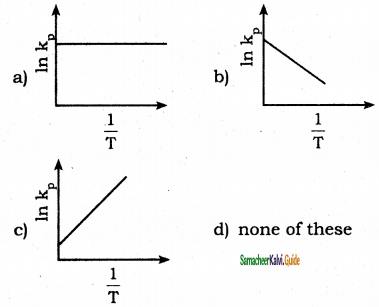b)Question 94.
The value of ∆G° for a reaction in aqueous phase having Kc = 1, would be
a) – RT
b) -1
c) 0
d) + RT
c) 0

Question 95.
The equilibrium constants for the reaction, Br2 ⇌ 2 Br at 500 K and 700 K are 1 × 10-10 and 1 × 10-5 respectively. The reaction is
a) endothermic
b) exothermic
c) fast
d) slow
a) endothermicQuestion 96.
In the reaction A2(g) + 4 B2(g) ⇌ 2AB4(g), ∆H < 0, the decomposition of AB4(g) will be favoured at
a) low temperature and high pressure
b) high temperature and low pressure
c) low temperature and low pressure
d) high temperature and high pressure
c) low temperature and low pressure

Question 97.
The equilibrium constant for the reaction N2(g) + O2(g) ⇌ 2NO (g) is 4 × 10-4 at 200 K. In the presence of a catalyst the equilibrium is attained 10 times faster. Therefore the equilibrium constant in pressure of the catalyst at 200 K is
a) 4 × 10-3
b) 4 × 10-4
c) 4 × 10-5
d) None
b) 4 × 10-4

Question 98.
Change in volume of the system does not alter the number of moles in which of the following equilibrium.
a) N2(g) + O2(g) ⇌ 2 NO (g)
b) PCl5(g) ⇌ PCl3(g) + Cl2(g)
c) N2(g) + 3H2(g) ⇌ 2 NH3(g)
d) SO2Cl2(g) ⇌ SO2(g) + Cl2(g)
a) N2(g) + O2(g) ⇌ 2NO2 (g)

Question 99.
In which of the following equilibrium reactions the equilibrium would shift to the right, if total pressure is increased
a) N2 + 3H2 ⇌ 2 NH3
b) H2 + I2 ⇌ 2HI
c) H2 + Cl2 ⇌ 2 HCl
d) N2O4 ⇌ 2NO
a) N2 + 3H2 ⇌ 2 NH3

Question 100.
The graph in K (eq) vs $$\frac{1}{T}$$ relates for a reaction must bea) Exothermic
b) Endothermic
c) ∆H is negligible
d) High spontaneous at ordinary temperature
a) Exothermic

Question 101.
Which of the following statements is cor¬rect for a reversible process in state of equilibrium?
a) ∆G° = -2.30 RT log K
b) ∆G° = 2.30 RT log K
c) ∆G = -2.30 RT log K
d) ∆G = 2.30 RT log K
a) ∆G° = -2.30 RT log K

Question 102.
Which of the following is a characteristic of a reversible reaction?
a) Number of moles of reactants and products are equal
b) it can be influenced by a catalyst
c) it can never proceed to completion
d) none of the above
c) it can never proceed to completion

Question 103.
The equilibrium constant in a reversible reaction at a given temperature
a) depends on the initial concentration of the reactants
b) depends on the concentration of the products at equilibrium
c) does not depend on the initial concentration
d) it is not characteristic of the reaction
c) does not depend on the initial concentrationII. Very short question and answers(2 Marks):

Question 1.
Define the state of equilibrium.
At a particular stage, the rate of the reverse reaction is equal to that of the forward reaction indicating a state of equilibrium.

Question 2.
What are irreversible reactions?
Reactions in which the products formed do not react to give back reactants under normal conditions are called irreversible reactions.

Question 3.
Why the chemical equilibrium is referred to as Dynamic Equilibrium?
At equilibrium, the forward and the backward reactions are proceeding at the same rate and no macroscopic change is observed. So the chemical equilibrium is often called dynamic equilibrium.

Question 4.
How is a gas – solution equilibrium exist?
When a gas dissolves in a liquid under a given pressure, there will be an equilibrium between gas molecuLes in the gaseous state and those dissolved in the liquid. Example – In carbonate beverages, the following equilibrium exists.
CO2(g) $$\rightleftharpoons$$  CO2 (Solution)

Question 5.
Write the Applications of the equilibrium constant?
The knowledge of the equilibrium constant helps us in

• predicting the extent of a reaction, predicting the direction in which the net reaction is taking place, and
• calculation equilibrium constants and concentration of the reactants and products.Question 6.
What is Le – Chatelier’s principle?
It states that “If a system at equilibrium is subjected to a change of concentration, pressure or temperature, then the equilibrium is shifted in such a way to nullify the effect of that disturbance.

Question 7.
For the H2(g) + I2(g) ⇌ 2HI(g) reaction the value of Kc at 717 K is 48. If at a particular instant, the concentration of H2, I2, and HI is 0.2 mol lit-1 and 0.6 mol lit-1 respectively, then calculate Q and predict the direction of the reaction.
Solution:
Q = $$\frac{\left[\mathrm{H}_{1}\right]^{2}}{\left[\mathrm{H}_{2}\right]\left[\mathrm{I}_{2}\right]}=\frac{0.6 \times 0.6}{0.2 \times 0.2}$$ = 9
Q < Kc, the reaction will proceed in the forward direction.

Question 8.
Define Degree of dissociation.
The fraction of the total number of moles of the reactants are dissociated.
Degree of dissociation ‘x’
‘x’ = $$\frac{\text { no.of moles dissociated }}{\text { Total no. of moles present initially }}$$

Question 9.
Dissociation of PCl5 decreases in presence of increase in Cl2, Why?

1. The dissociation equilibrium of PCl5 in gaseous state is written as PCl5(g) ⇌ PCl3(g) + Cl2(g)
2. In a chemical equilibrium increasing the concentration of the products results in shifting the equilibrium in favour of the reactants. So in the presence of increased Cl2 reversed reaction only favoured.
3. Hence dissociation of PCl5 decreases in presence of increase in Cl2.

Question 10.
The equilibrium constant for Kc for A(g) ⇌ B(g) is 2.5 × 10-2 . The rate constant of the forward reaction is 0.05 sec-1. Calculate the rate constant of the reverse reaction.
Kc = 2.5 × 10-2;
Kf = 0.05 sec-1
Kc = $$\frac{\mathrm{K}_{\mathrm{f}}}{\mathrm{K}_{\mathrm{r}}}$$
= $$\frac{0.05}{2.5 \times 10^{-2}}$$ = 2
Rate constant of the reverse reaction,
Kr = 2 sec-1.Question 11.
The equilibrium constant for the reaction 2SO3(g) ⇌ 2SO2(g) + O2(g) is 0.15 mol dm-3 at 900K. Calculate the equilibrium constant for the reaction 2SO2(g) + O2(g) ⇌ 2SO3(g) at the same temperature. 2SO3(g) ⇌ 2SO2(g) + O2(g).
Kc = $$\frac{\left[\mathrm{SO}_{2}\right]^{2}\left[\mathrm{O}_{2}\right]}{\left[\mathrm{SO}_{3}\right]^{2}}$$ = 0.15
K’ = $$\frac{1}{\mathrm{~K}_{\mathrm{c}}}=\frac{1}{0.15}$$
= 6.6 mole dm-3
K’ = 6.6 mole dm-3

III. Short question and answers (3 Marks):

Question 1.
Explain about the extent of reaction of dissociation of bromine mono chloride at 1000 K.
2BrCl(g) $$\rightleftharpoons$$ Br2(g) + Cl2(g) KC = 5
10-3 <  KC <  103
both forward and backward reaction make significant progress. Neither forward nor reverse reaction predominates.

Question 2.
What is liquid – vapour equilibrium?
The equilibrium of a liquid with its gas at its boiling point at 1 atm pressure is called liquid-vapour equilibrium.
Rate of evaporation = Rate of condensation
H2O(l) ⇌ H2O(g).

Question 3.
What is Solid – vapour equilibrium?

• When solid iodine is placed in a closed transparent vessel, after some time the vessel gets filled up with violet vapour.
• The intensity of the colour increases and finally becomes constant, i.e., equilibrium is attained.
I2(s) ⇌ I2(g)

Question 4.
What are Homogeneous and Heterogeneous equilibriums?
Homogeneous equilibrium:
In a homogeneous equilibrium, all the reactants and products are in the same phase.
Example:
H2(g) + I2(g) ⇌ 2HI (g)
In the above equilibrium, H2, I2, and HI are in the gaseous state.
Similarly, for the following reactions,
CH3COOCH3(aq) + H2O(aq) ⇌ CH3COOH (aq) + CH3OH (aq) all the reactants and products are in homogenous solution phase.

Heterogeneous equilibrium:
If the reactants and products are present in different phases, it is known as heterogeneous equilibrium.
Example:
H2O(l) ⇌ H2O (g)
CaCO3(s) ⇌ CaO(s) + CO2(g).

Question 5.
Define Q value for a chemical equilibrium reaction.
Consider a homogeneous reversible reaction xA + yB $$\rightleftharpoons$$ lC + mD For the above reaction under non-equilibrium conditions, reaction quotient Q is defined as the ratio of the product of active masses of reaction products raised to the respective stoichiometric coefficients in the balanced chemical equation to that of the reactants. Under non-equilibrium conditions,
Q = $$\frac { d(Ink) }{ dt }$$ ΔH° = $$\frac { { \triangle H }^{ 0 } }{ { RT }^{ 2 } }$$Question 6.
What is the reaction quotient? Write the relation of K & Q.
Reaction quotient ‘Q’ is defined as the ratio of the product of active mass of reaction products raised to the respective stoichiometric coefficients in the balanced chemical equation to that of the reactants under non equilibrium.
Reaction quotient Q.
Q = $$\frac{[\mathrm{C}]^{1}[\mathrm{D}]^{\mathrm{m}}}{[\mathrm{A}]^{\mathrm{x}}[\mathrm{B}]^{\mathrm{y}}}$$
If Q = Kc, the reaction is in equilibrium
If Q > Kc, the reaction will proceed in the reverse direction i.e., formation of reactants.
If Q < Kc, the reaction will proceed in the forward direction i.e., formation of products.

Question 7.
Explain effect of pressure of formation of HI.
Pressure has no effect on the equilibrium when the total number of the moles of the gaseous reactants and the gaseous products are equal. ( ∆ng = 0).
Therefore, pressure has effect only on the equilibrium with ∆ng = 0.
Let us consider the following reactions
H2(g) + I2(g) ⇌ 2HI(g)
N2(g) + O2(g) ⇌ 2NO(g)
Here,
The number of moles on reactants and products are equal. So pressure has no effect on them.

Question 8.
The equilibrium concentrations of NH3, N2 and H2 are 1.8 × 10-2 M, 1.2 × 10-2 M and 3 × 10-2 M respectively. Calculate the equilibrium constant for the formation of NH3 from N2 and H2. [Hint: M= mol lit-1]
Solution:
Given data:
[NH3] = 1.8 × 10-2 M ;
[N2] = 1.2 × 10-2 M ;
[H2] = 3 × 10-2 M ;
Kc = ?
N2(g) + 3H2(g) ⇌ 2NH3(g)
Kc = $$\frac{\left[\mathrm{NH}_{3}\right]^{2}}{\left[\mathrm{~N}_{2}\right]\left[\mathrm{H}_{2}\right]^{3}}$$
Kc = $$\frac{1.8 \times 10^{-2} \times 1.8 \times 10^{-2}}{1.2 \times 10^{-2} \times 3 \times 10^{-2} \times 3 \times 10^{-2} \times 3 \times 10^{-2}}$$

Kc = 1 × 10-2 l2 mol-2

Question 9.
One mole of H2 and one mole of I2 are allowed to attain equilibrium. If the equilibrium mixture contains 0.4 mole of HI. Calculate the equilibrium constant.
Given data:
[H2] = 1 mole;
[I2] = 1 mole
At equilibrium, [HI] = 0.4 mole; Kc = ?
H2(g) + I2 ⇌ 2HI(g)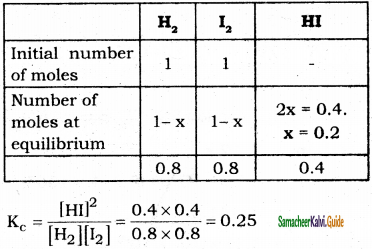Question 10.
Relate the three equilibrium constants.
i) N2 + O2 ⇌ 2NO; K1
ii) 2NO + O2 ⇌ 2NO2; K2
iii) N2 + 2O2 ⇌ 2NO2; K3Question 11.
Write the equilibrium constant for the following:
i)H2O2(g) ⇌ H2O + 1/2O2(g)
ii) CO(g) + H2O(g) ⇌ CO2(g) + H2(g)
iii) N2O4(g) ⇌ 2NO2(g)
i)H2O2(g) ⇌ H2O + 1/2O2(g)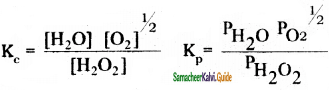ii) CO(g) + H2O(g) ⇌ CO2(g) + H2(g)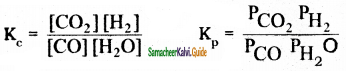iii) N2O4(g) ⇌ 2NO2(g)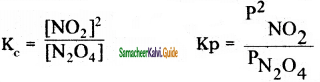Question 12.
Calculate the ∆ng, for the following reactions:
i) H2(g) + I2(g) ⇌ 2HI
ii) 2H2O(g) + 2Cl2(g) ⇌ 4HCl(g) + O2(g)
∆ng = (Total number of moles of gaseous products) – (Total number of moles of gaseous reactants)
i) H2(g) + I2(g) ⇌ 2HI
∆ng = 2 – 2 = 0
∆ng = 0

ii) 2H2O(g) + 2Cl2(g) ⇌ 4HCl(g) + O2(g)
∆ng = 5 – 4 = 1
∆ng = 1Question 13.
For the reaction A+B ⇌ 3C at 25°C, a 3 litre volume reaction vessel contains 1, 2, and 4 moles of A, B, and C respectively at equilibrium, calculate the equilibrium constant Kc of the reaction at 25°C.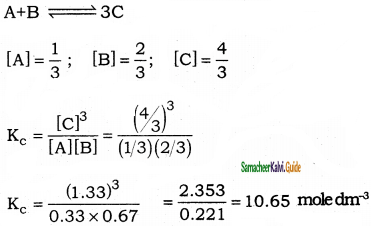Question 14.
At temperature T1 the equilibrium constant of reaction is K1 At a higher temperature T2, K2 is 10% of K1. Predict whether the equilibrium is endothermic or exothermic.
At temperature, T1, the equilibrium constant is K1
At a high temperature, T2, the equilibrium constant K2 = 10%KX
K2 = $$\frac{10}{100}$$ K1
K2 = 0.1 K1
K1 > K2
Hence, the equilibrium is exothermic.

Question 15.
Calculate the Kc when a mixture containing 8.07 moles of H2 and 9.08 moles of I2 are reacted at 448°C until 13.38 moles of HI was formed at the equilibrIum.
The equilibrium reaction is,
H2(g) + I2(g) ⇌ 2HI(g)
Kc = $$\frac{(2 x)^{2}}{(a-x)(b-x)}$$
a = 8.07 moles;
b = 9.08 moles
2x = 13.38 moles
x = 6.69 moles
Kc = $$\frac{4(6.69)^{2}}{(8.07-6.69)(9.08-6.69)}$$

= $$\frac{4 \times 44.75}{1.38 \times 2.39}$$
Kc = 54.29

Question 16.
For the equilibrium 2NOCl(g) ⇌ 2NO(g) + Cl2(g) the value of the equilibrium constant Kc is 3.75 × 10-6 at 790°C. Calculate Kp for this equilibrium at the same temperature. [Hint: Kp = Kc(RT)∆ng]
Kc = 3.75 × 10-6;
T = 790 + 273 = 1063 K
Kp = Kc × (RT)∆ng
For the reaction,
2NOCl(g) ⇌ 2NO(g) + Cl2(g)
∆ng = 3 – 2 = 1
Kp = 3.75 × 10-6 × (8.314 × 1063)
= 33141.6 × 10-6
= 3.31 × 104 × 10-6
Kp = 3.31 × 10-2

Question 17.
How much PCl5 must be added to one litre volume reaction vessel at 250°C in order to obtain a concentration of 0.1 mole of Cl2, Kc for PCl5 ⇌ PCl3 + Cl2 is 0.0414 mol dm-3 at 250°C.
PCl5 ⇌ PCl3 + Cl2
Number of moles of PCl5 at equilibrium = a – 0.1
Number of moles PCl3 at equilibrium = 0.1
Number of moles Cl2 at equilibrium = 0.1
Kc = $$\frac{\left[\mathrm{PCl}_{3}\right]\left[\mathrm{Cl}_{2}\right]}{\left[\mathrm{PCl}_{5}\right]}$$
= 0.0414
= $$\frac{0.1 \times 0.1}{a-0.1}$$
0.01 = (a – 0.1) 0.0414
0.01 = 0.0414a – 0.00414
0.0414a = 0.01414
a = 0.3415 mole

Question 18.
At 540 K, the equlllbrium constant Kp for PCl5 dissociation equilibrium at 1.0 atm is 1.77 atm. Calculate equilibrium constant in molar concentration (Kc) at same temperature and pressure.
Kp = 1.77 atm;
T = 540 K;
a = 0.082
PCl5 ⇌ PCl3 + Cl2
∆ng = 2 – 1 = 1
Kp = Kc(RT)∆ng
1.77 = Kc × (0.0821 × 450)1
Kc = $$\frac{1.77}{(0.0821 \times 540)}$$
= 4 × 10-2 moles/litres.III. Long question and answers (5 Marks):

Question 1.
Explain the formation of solid-liquid equilibrium with a suitable example.
1. Consider melting of ice in a closed container at 273 K. This system reaches a state of physical equilibrium in which the amount of water in the solid phase and liquid phase does not change with time.

2. In this process. the total number of water molecules leaving from and returning to the solid phase at any instant are equal.

3. If some ice cubes and water are placed in a thermos flask (at 273K and I atm) then there will be no change in the mass of ice and water.

4. At equilibrium: Rate of melting of ice Rate of freezing of water
H2O(l) $$\rightleftharpoons$$ H2O(g)

Question 2.
Derive Kp & Kc relation for the formation of HI.
Synthesis of HI:
Consider that ‘a’ moles and ‘b’ moles are the initial concentration of H2 and I2 respectively, taken in a container of volume V. Let ‘x’ moles of each of H2 and I2 react together to form 2x moles of HI.
H2(g) + I2(g) ⇌ 2HI(g)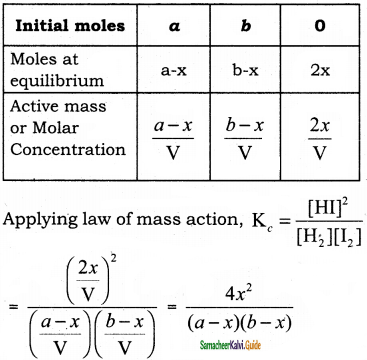The equilibrium constant Kp can also be calculated as follows:
Total number of moles at equilibrium = a – x + b – x + 2x = a + b.
If the total pressure of the system at equilibrium be P, then
Partial pressure of H2,
pH2 = $$\left(\frac{a-x}{a+b}\right)$$P

Partial pressure of I2,
pI2 = $$\left(\frac{b-x}{a+b}\right)$$P

Partial pressure of HI,
pHI = $$\left(\frac{2x}{a+b}\right)$$P

Kp = $$\frac{\left(\frac{2 x}{a+b}\right)^{2} \mathrm{P}^{2}}{\left(\frac{a-x}{a+b}\right) \mathrm{P}\left(\frac{b-x}{a+b}\right) \mathrm{P}}=\frac{4 x^{2}}{(a-x)(b-x)}$$
In the above equilibrium, Kc and Kp are thus shown to be equal.Question 3.
Derive the equilibrium constant Kp & Kc for the Dissociation of PCl5.
Dissociation of PCl5:
Consider that ‘a’ moles is the initial concentration of PCl5 taken in a container of volume V. Let ‘x’ moles of PCl5 be dissociated into x moles each of PCl3 and Cl2.
PCl5(g) ⇌ PCl3(g) + PCl2(g)The equilibrium constant Kp can also be calculated as follows:
Total number of moles at equilibrium = a – x + x + x = a + x
If the total pressure of the system at equilibrium be P, then
Partial pressure of PCl5,
pPCl5 = $$\left(\frac{a-x}{a+x}\right)$$P;

Partial pressure of PCl3,
pPCl3 = $$\left(\frac{x}{a+x}\right)$$P;

Partial pressure of Cl2
pCl2 = $$\left(\frac{x}{a+x}\right)$$P

Kp = $$\frac{\left(\frac{x}{a+x}\right) \mathrm{P}\left(\frac{x}{a+x}\right) \mathrm{P}}{\left(\frac{a-x}{a+x}\right) \mathrm{P}}=\frac{x^{2} \mathrm{P}}{(a-x)(a+x)}$$

Question 4.
Explain the effect of Concentration of formation of HI?
Effect of concentration:
At equilibrium, the concentration of the reactants and the products does not change. The addition of more reactants or products to the reacting system at equilibrium causes an increase in their respective concentrations.

According to Le Chatelier’s principle, the effect of increase in concentration of a substance is to shift the equilibrium in a direction that consumes the added substance. Suppose at equilibrium if one of the reactants is added, then the equilibrium will shift in the forward direction and if one of the products is added, then the equilibrium will shift in backward direction.
Let us consider the reaction,
H2(g) + I2(g) ⇌ 2HI(g)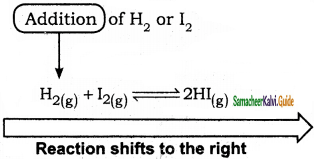On addition of H2 or I2 to the equilibrium mixture product HI will tend to increase. In order to minimize the stress, the system shifts the reaction in a direction where H2 and I2 are consumed i.e., the formation of HI would relieve the stress. Hence, the equilibrium shifts to the right (forward direction).

Similarly, removal of HI (product) also boosts the forward reaction and increases the concentration of products.
If HI is added to the equilibrium mixture, the concentration HI is increased, then the system proceeds in the reverse direction to nullify the stress.

Question 5.
The equilibrium constant at 298 K for a reaction is 100. A + B ⇌ C + D. If the initial concentration of all the four species were 1 M each what will be equilibrium concentration of D (in mol lit-1)
Solution:
Given data:
[A] = [B] = [C] = 1 M;
Kc = 100; [D] = ?10(1 – x) = 1 + x
10 – 10x – 1 – x = 0
9 – 11x = 0
11x = 9
x = 9/11 = 0.818
So, [D] at equilibrium = 1 + 0.818 = 1.818 MQuestion 6.
What happens when ∆ng = 0, ∆ng = -ve, ∆ng= +ve In a gaseous reaction?
(i) When ∆ng = 0, the total number of moles of gaseous products are equal to the total number of moles of gaseous reactants.
Eg:
H2(g) + I2(g) ⇌ 2HI(g)
∆ng = 2 – 2 = 0
Kp = Kc(RT)∆ng
If ∆ng = 0,
Kp = Kc(RT)0
∴ Kp = Kc

(ii) When ∆ng = +ve, the total number of moles of gaseous products are greater than the total number of moles of gaseous reactants.
Eg:
2H2O + 2Cl2(g) ⇌ 4HCl(g) + O2(g)
∆ng = 5 – 4 = 1
Kp = Kc(RT)1
Kp = Kc(RT)
∴ Kp > Kc

(iii) When ∆ng = -ve, the total number of moles of gaseous products are greater than the total number of moles of gaseous reactants.
N2(g) + 3H2(g) ⇌ 2NH3(g)
∆ng = 2 – 4 = -2
Kp = Kc(RT)-2

Kp = $$\frac{K_{c}}{(R T)^{2}}$$

∴ Kp < Kc

Question 7.
What is the relationship between the dissociation and formation equilibrium constant?
In a chemical equilibrium reaction, the equilibrium constant for the dissociation equilibrium reaction which is also known as the dissociation constant is found to be the reciprocal value of the equilibrium constant for the formation equilibrium reaction.
Formation of equilibrium reaction,
2SO2(g) + O2 ⇌ 2SO3
Kc = $$\frac{\left[\mathrm{SO}_{3}\right]^{2}}{\left[\mathrm{SO}_{2}\right]^{2}\left[\mathrm{O}_{2}\right]}$$ dm3 /mole.

Dissociation equilibrium reaction of SO3 the reactants became products and vice versa.
2SO3(g) ⇌ 2SO2(g) + O2(g)
Kc‘ = $$\frac{\left[\mathrm{SO}_{2}\right]^{2}\left[\mathrm{O}_{2}\right]}{\left[\mathrm{SO}_{3}\right]^{2}}$$ mole/dm3
= $$\frac{1}{\mathrm{~K}_{\mathrm{c}}}$$

Kc‘ is considered as the dissociation constant of SO3 gas. Usually, the equilibrium constant of the dissociation equilibrium is the reciprocal of the equilibrium constant of the formation equilibrium reaction.

Question 8.
In the equilibrium H2 + I2 ⇌ 2HI, the number of moles of H2, I2, and HI are 1, 2, 3 moles respectively. Total pressure of the reaction mixture is 60 atm. Calculate the partial pressures of H2, I2, and HI in the mixture.
Solution:
H2 + I2 ⇌ 2HI
Number of moles of H2 at equilibrium = 1 mole
Number of moles of I2 at equilibrium = 2 mole
Number of moles of HI at equilibrium = 3 mole
Total number of moles of equilibrium = 1 + 2 + 3 = 6 moles
Mole fraction of H2 = 1/6;
Mole fraction of I2 = 2/6;
Mole fraction of HI = 3/6
Partial pressure = Mole fraction × Total pressure
PH2 = $$\frac{1}{6}$$ × 60 = 10 atm;
PI2 = $$\frac{2}{6}$$ × 60 = 20 atm;
PHI = $$\frac{3}{6}$$ × 60 = 30 atm.Question 9.
In 1 litre volume reaction vessel, the equilibrium constant K of the reaction PCl5 ⇌ PCl3 + Cl2 is 2 × 10-4 What will be the degree of dissociation assuming only a small extent of 1 mole of PCl5 has dissociated?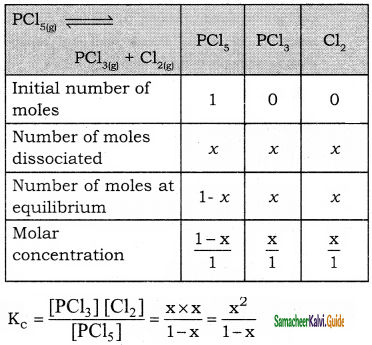since ‘x’ is very small compared to 1,
then K2 = x2
2 × 10-4 = x2
Degree of dissociation x = $$\sqrt{2 \times 10^{-4}}$$
= 1.414 × 10-2Question 10.
At 35°C, the value of K for the equilibrium reaction N2O4 ⇌ 2NO2 is 0.3174, Calculate the degree of dissociation when P is 0.2382 atm.

 N2O4 ⇌ 2NO2 N2O4 NO2 The initial number of moles 1 0 Number of moles dissociated x 2x Number of moles  equilibrium 1 – x 2x

Total number of moles at equilibrium = 1 – x + 2x = 1 + xQuestion 11.
Degree of dissociation of PCl5 at 1 atm and 25°C is 0.2. Calculate the pressure at which PCl5 is half dissociated at 25°C.
For PCl5 dissociation equilibrium,
Kp = $$\frac{x^{2} p}{1-x^{2}}$$ [ P = total pressure = 1 atm]
x = 0.2
Kp = $$\frac{(0.2)^{2}(1.0)}{1-(0.2)^{2}}$$
= $$\frac{0.04}{1-0.04}$$Question 12.
Initially, 0.1 moles each of H2 and I2 gases and 0.02 moles of HI gas are mixed in a reaction vessel of constant volume at 300K. Predict the direction towards which the reaction proceeds [K = 3.5 x 10-2].
Kc = $$\frac{[\mathrm{HI}]_{\mathrm{e}}^{2}}{\left[\mathrm{H}_{2}\right]_{\mathrm{e}}\left[\mathrm{I}_{2}\right]_{\mathrm{e}}}$$
Q = $$\frac{[\mathrm{HI}]^{2}}{\left[\mathrm{H}_{2}\right]\left[\mathrm{I}_{2}\right]}$$
= $$\frac{\left(2 \times 10^{-2}\right)^{2}}{0.1 \times 0.1}$$